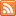Content Tagged “Conditional Probability Rule (Rule #7)”Conditional Probability and Independence

Published: Sep 19th, 2013

View Lecture Slides with Transcript – Conditional Probability and Independence Part 1 (14:55) Slides 1-11 Part 2 (13:18) Slides 12-18 This document is linked from Conditional Probability and Independence.

Interactive Applet – Conditional Probability

Published: Jan 28th, 2013

Now that we have introduced conditional probability concepts, try this interactive demonstration which uses Venn diagrams to illustrate the probabilities we have been discussing. You can choose which event to shade and […]

Did I Get This – Conditional Probability

Published: Jan 28th, 2013

Consider the piercing example, where the following two-way table is given, Recall also that M represents the event of being a male (“not M” represents being a female), and E […]Conditional Probability and Independence

Published: Jul 28th, 2012

Independent Events Multiplication Rule for Independent Events (Rule Six) Conditional Probability (Rule Seven) Independent Events (Part 2) General Multiplication Rule (Rule Eight) Let’s Summarize CO-6: Apply basic concepts of probability, random […]